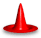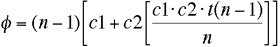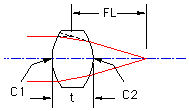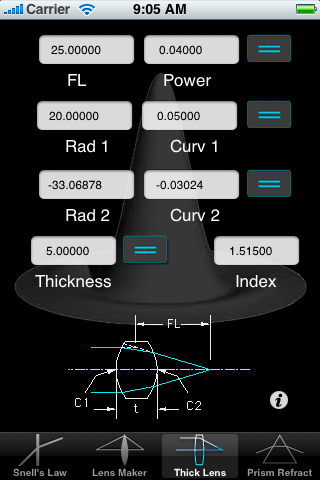OpticsCalc for iPhone: Paraxial Thick LensDescriptionThe Paraxial Thick Lens pane computes the first order properties of a paraxial thick lens in air. The equation for the power (1/fl) of a paraxial thick lens is given by the equation shown below.Where:
c1 - curvature of surface 1
c2 - curvature of surface 2
n - index of refraction of lens material
t - center thickness of lens.Those who are not familiar with optics should realize that the focal length is not the distance from the second surface of the lens to the focal point, but rather the distance from the point where a ray entering the lens parallel to the optical axis would intersect the refracted ray if they were both extended towards that intersection point. For a single element lens, this point commonly lies between the two planes perpendicular to the optical axis located where the surfaces intersect the axis.

You must enter the index of refraction and any three of the other 4 values. Once you fill in the known quantities, select the value you want to calculate by pressing the equal sign. Note that you are allowed to enter either the value (focal length, Radius 1 and Radius 2) or their inverse. After you enter the value, the inverse is computed upon pressing the return key.A Note On UnitsUnits are whatever the user wants, but they must be the same for all values (except of course for index which is unitless).